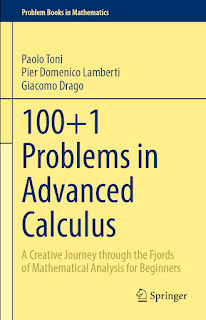CALCULUS MATHEMATICS

# 100+1 Problems in Advanced Calculus A Creative Journey through the Fjords of Mathematical Analysis for Beginners PDF Free DownloadThis book convenes a collection of carefully selected problems in mathematical analysis, crafted to achieve maximum synergy between analytic geometry and algebra and favoring mathematical creativity in contrast to mere repetitive techniques. With eight chapters, this work guides the student through the basic principles of the subject, with a level of complexity that requires good use of imagination. In this work, all the fundamental concepts seen in a first-year Calculus course are covered. Problems touch on topics like inequalities, elementary point-set topology, limits of real-valued functions, differentiation, classical theorems of differential calculus (Rolle, Lagrange, Cauchy, and l’Hospital), graphs of functions, and Riemann integrals and antiderivatives. Every chapter starts with a theoretical background, in which relevant definitions and theorems are provided; then, related problems are presented. Formalism is kept at a minimum, and solutions can be found at the end of each chapter.